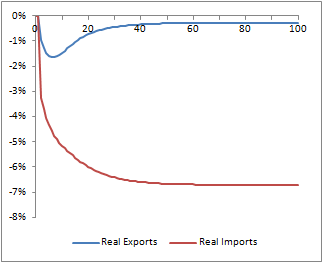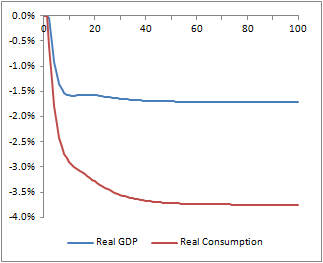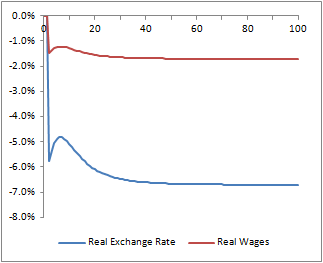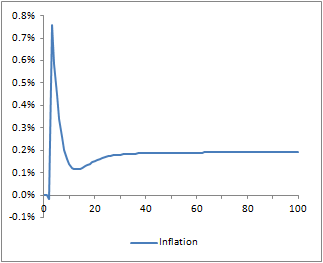Wednesday, 6 July 2016

An SFC Analysis of a Shock to Export Capability

The UK's decision to quit the EU has left a lot of uncertainty over its future trading position.  It will probably be a long time before it becomes clear what kind of arrangements can be negotiated, but the concern is that the UK could suffer damage to export capability, with little reduction in its susceptibility to import penetration.

One immediate consequence of these concerns has been a sharp fall in sterling.  In principle, this should be good news for UK export capability, and there was a case to be made that sterling was overvalued before the referendum.  However, if this indeed reflects a weakened trade position, then assessing the overall impact is more tricky.

As such, I thought it would be interesting to use a stock-flow consistent model to look at how a shock to export capability and consequent exchange rate weakening might impact on overall activity.

The model I have used here is essentially the same as one described in an earlier post.  It is purely conceptual - no attempt has been made to calibrate it to the UK.  The main difference compared to the earlier version is that I have expanded on the way inflation is determined.  The mechanism here is based on real wage targeting and follows that used in Godley & Lavoie (p 302), incorporating adaptive inflation expectations.  Pricing is a straightforward mark-up to unit labour costs.

Otherwise, the only real departure from what might be found in Godley and Lavoie is the use of forward looking expectations for the exchange rate.  This is useful here, where we want to look at the impact of a change in expectations of future trade prospects.

As usual, I have provided a full equation listing at the end of the post.

The graphs below show the effect on selected variables of a 5% fall in the base level of exports (i.e. before taking account of changes due to relative price).One of the first things to note is that although this leads to a fall in actual real exports, there is a much greater fall in real imports.  This is due to the fall in the real exchange rate.  Exports become cheaper which mitigates the impact of the change in the base level of demand.  However, this worsening in the terms of trade means that the level of foreign earnings from exporting has fallen dramatically.  The amount of imports this buys has been significantly reduced.

In itself, the fact that import volumes have fallen by more than export volumes might be expected to provide a boost to domestic production.  However, the worsening of the terms of trade reduces the real value of domestic income, specifically when we measure by reference to the consumer price deflator rather than the GDP deflator.  Consider what happens to the real wage as shown in the graph.  The nominal wage deflated by the price of domestic output is a constant, because of the mark-up pricing.  But, because consumption also includes imports, when the real wage is deflated by the consumer price index it closely reflects changes in the real exchange rate.

This erosion in the value of real income reduces consumer expenditure and this is enough to more than outweigh the boost to output from reduced imports.

Although the scale of this depends on the parameters, the direction of change is a consequence of the stock-flow structure.  In a model like this, GDP tends towards a level which achieves a steady state level of government debt.  This is largely determined by the fiscal stance.  However, inflation will have an impact because it erodes the real value of the government debt.

The decline in the real exchange rate gives an inflationary impulse.  Eventually, this settles down to a higher level of inflation giving a reduced real return on domestic assets compared with overseas assets.  This is necessary because, when valued in the same currency, the fall in the real exchange rate has made the supply of domestic assets lower relative to the supply of foreign assets. (It is, of course, possible that seperate factors might also impact on the relative demand for these assets.  This is not considered here, but is obviously relevant in the context of the fallout from the Brexit vote.)

The higher level of inflation can be seen in graph.  This erodes government debt, requiring the state to run a slightly higher deficit to compensate.  This higher deficit implies lower tax revenues, which in turn implies a lower level of GDP.

Equation Listing

Equations (1) to (15) are as described in the previous model.

(1)        y =  d + g + x

(2)        d = ( C - m . pf / e ) / p

(3)        C = α1 . YD  + α2 . ( Bd-1 + Fd-1 / e )

(4)        YD = y . p - T + r-1 . BD-1 + rf . Fd-1 / e

(5)        T = τ .  ( y . p + r-1 . Bd-1 + rf . Fd-1 / e )

(6)        m = d . µ . ( e . p / pf )σ1

(7)        x = x0 . ( e . p / pf )σ2

(8)        V = YD - C + Bd-1 + Fd-1 / e

(9)        Fd = e . λ1 . V. ( rrf / r )κ1

(10)      Bw = λ2 . Vf / e . ( rrd / rf )κ2

(11)      B = B-1 . ( 1 +r-1 ) + g . p - T

(12)      Bd = V - Fd / e

(13)      Bw = B - Bd

(14)      rrd = ( 1 + rd ) . E[ e+1 ] / e - 1

(15)      rrf = ( 1 + rf ) . e / E[ e+1 ] - 1

Employment is proportional to output.

(16)      n = φ . y

The target real wage is based on the level of employment.

(17)      wrt = wrt0 . ( n / n0 )δ

Nominal wage adjustment is based on inflation expectations and the gap between the target real wage and the previous actual real wage.

(18)      w = w-1 . E[π] . ( wrt . pc-1  / w-1 )ψ

Prices are a straight mark-up to unit labour costs.

(19)      p = β . w . n / y

Consumer prices are approximated as the deflator between nominal consumer spending and an aggregate volume measure.

(20)      pc = C / ( d + m )

(21)      E[π] = E[π]-1 + ε . ( π-1 - E[π]-1 )

Where inflation is taken as the change in consumer prices (expressed as 1 plus the change).

(22)      π = pc / pc-1

Variables

The variables are listed below.  Uppercase variables denote nominal values.

 B Domestic bonds Bd Domestic bonds held by domestic investors Bw Domestic bonds held by overseas investors d Domestic expenditure by the domestic private sector e Exchange rate (units of foreign currency per unit of domestic currency) Fd Overseas bonds held by domestic investors (in foreign currency terms) g Real government expenditure m Real imports n Employment n0 Base level of employment p Price of domestic output pc Consumer price deflator pf Price of foreign output r Interest rate on domestic bonds rf Interest rate on foreign bonds rrd Expected return to foreign investors on domestic bonds rrf Expected return to domestic investors on foreign bonds T Taxes V Net financial wealth of domestic private sector Vf Financial wealth of rest of world (in foreign currency terms) w Nominal wage wrt Target real wage wrt0 Base level of target real wage x Real exports x0 Base level of real exports y Real output YD Disposable income of domestic private sector π Consumer price inflation

Policy variables are the level of government expenditure, the tax rate (τ) and the domestic interest rate.  Foreign variables (Vf, x0 and rf) are taken as exogenous in accordance with the "small" economy assumption.  E[  ] denotes the expected value of a variable.  Expected values of exchange rates are set equal to actual outcomes, except for the period of the shock.